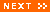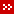The average diameter of a red blood cell is 7 x 10-6 m. (0.000007m)The distance from the Earth to the Sun is 1.496 x 1011m (149,600,000,000m)To use scientific notation successfully you need to remember that:

• 102 = 100, 103 = 1000, 104 = 10,000 and so on - and also that:
• 10-1 =1/10 = 0.1, 10-2 = 1/100= 0.01, 10-3 = 1/1000= 0.001 and so on.

A tip to remember is to count the places for zeroes.

• In the speed of light example 3,00,000,000 there are eight between the leftmost number and the decimal point - 3 x 108m/s.

When counting places for zeroes with very small numbers you need to include the zero to the left of the decimal point too.

• In the red blood cell example, diameter is 0.000007m  - 7 x 10-6 m.Significant FiguresAverage ratingNot yet ratedNo comments yet. Why not be the first person to add one?
Bookmark this pageIf you want to practise converting very small numbers to scientific notation try this websiteAAA Math